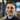BlogProjects

# Posgres per-table autovacuum management

02 December 2020

You need to tune Postgres auto-vacuum settings, but per-table can be hard to manage.

Postgres auto-vacuum and auto-analyze settings are important for managing Postgres performance. The first improvement you will probably make is adjusting the default, and conservative global values. These values will generally work for smaller data sizes but will start causing pain in larger data sets. The trigger kicks in using `(scale_factor * rows) + threshold`, so for large tables a default scale_factor of `0.20` will trigger far less often than you may like.

### Default global auto-vacuum settings

``````autovacuum_analyze_scale_factor = .10
autovacuum_analyze_threshold = 50
autovacuum_vacuum_scale_factor = .20
autovacuum_vacuum_threshold = 50``````

After changing global settings, you will likely have tables with significantly different characteristics than others. This leads us into per-table tuning, which can be pretty easily

### Alter table-specific autovacuum settings

``ALTER TABLE foo SET (autovacuum_vacuum_scale_factor = 0, autovacuum_vacuum_threshold = 100)``

While making the changes on one table might be a good start, it is easy to start losing track of which tables have what settings. For myself, it is nice to have a quick view of what the current settings are.

This is a query that gives all the high-level information to understand the status of your per-table auto-vacuum settings:

column notes
nspname the namespace of the table
relname the table name
reltuples total number of tuples
n_dead_tup number of dead tuples needing to be vacuumed
v_threshold autovacuum threshold for dead tuples
n_mod_since_analyze number of tuples modified since last analyze
a_threshold autoanalyze threshold for dead tuples
caf custom autoanalyze factor
cat custom autoanalyze threshold
cvt custom autovacuum threshold
cvf custom autoanalyze factor
af global autoanalyze factor
at gloval autovacuum threshold
vf global autovacuum factor
vt global autovacuum threshold
last_vacuum
last_autovacuum

### View All table-specific auto-vacuum settings

``````WITH raw_data AS (
SELECT
pg_namespace.nspname,
pg_class.relname,
pg_class.oid AS relid,
pg_class.reltuples,
pg_stat_all_tables.n_dead_tup,
pg_stat_all_tables.n_mod_since_analyze,
(SELECT split_part(x, '=', 2) FROM unnest(pg_class.reloptions) q (x) WHERE x ~ '^autovacuum_analyze_scale_factor=' ) as c_analyze_factor,
(SELECT split_part(x, '=', 2) FROM unnest(pg_class.reloptions) q (x) WHERE x ~ '^autovacuum_analyze_threshold=' ) as c_analyze_threshold,
(SELECT split_part(x, '=', 2) FROM unnest(pg_class.reloptions) q (x) WHERE x ~ '^autovacuum_vacuum_scale_factor=' ) as c_vacuum_factor,
(SELECT split_part(x, '=', 2) FROM unnest(pg_class.reloptions) q (x) WHERE x ~ '^autovacuum_vacuum_threshold=' ) as c_vacuum_threshold,
to_char(pg_stat_all_tables.last_vacuum, 'YYYY-MM-DD HH24:MI:SS') as last_vacuum,
to_char(pg_stat_all_tables.last_autovacuum, 'YYYY-MM-DD HH24:MI:SS') as last_autovacuum
FROM
pg_class
JOIN pg_namespace ON pg_class.relnamespace = pg_namespace.oid
LEFT OUTER JOIN pg_stat_all_tables ON pg_class.oid = pg_stat_all_tables.relid
WHERE
n_dead_tup IS NOT NULL
AND nspname NOT IN ('information_schema', 'pg_catalog')
AND nspname NOT LIKE 'pg_toast%'
AND pg_class.relkind = 'r'
), data AS (
SELECT
*,
COALESCE(raw_data.c_analyze_factor, current_setting('autovacuum_analyze_scale_factor'))::float8 AS analyze_factor,
COALESCE(raw_data.c_analyze_threshold, current_setting('autovacuum_analyze_threshold'))::float8 AS analyze_threshold,
COALESCE(raw_data.c_vacuum_factor, current_setting('autovacuum_vacuum_scale_factor'))::float8 AS vacuum_factor,
COALESCE(raw_data.c_vacuum_threshold, current_setting('autovacuum_vacuum_threshold'))::float8 AS vacuum_threshold
FROM raw_data
)
SELECT
relid,
nspname,
relname,
reltuples,
n_dead_tup,
ROUND(reltuples * vacuum_factor + vacuum_threshold) AS v_threshold,
n_mod_since_analyze,
ROUND(reltuples * analyze_factor + analyze_threshold) AS a_threshold,
c_analyze_factor as caf,
c_analyze_threshold as cat,
c_vacuum_factor as cvf,
c_vacuum_threshold as cvt,
analyze_factor as af,
analyze_threshold as at,
vacuum_factor as vf,
vacuum_threshold as vt,
last_vacuum,
last_autovacuum
FROM
data
ORDER BY n_dead_tup DESC;``````

We pull a few columns from the pg_class table, which is the catalog for anything that looks like a table. The `relkind` field narrows our search down to an ‘ordinary table’. As well as filter out the informationschema, pgcatalog and anything that looks like a toast table with pg_toast.

The pgstatall_tables records table statistics, which gives us the number of dead tuples in a table.

To get the namespace name we join the pg_namespace table.

It is worth noting that `reloptions`, the field for custom table auto-vacuum settings, is a string array. While most often its vacuum and analyze settings that are configured on a per-table basis, you could modify this query to view any of the other vacuum tuning options like `autovacuum_vacuum_cost_limit` if those are being used.Written by Andrew Klotz. Building tools to help people to do their best. You should follow him on Twitter